Courses

# Civil Engineering (CE) Notes | EduRev

## Civil Engineering (CE) : Civil Engineering (CE) Notes | EduRev

The document Civil Engineering (CE) Notes | EduRev is a part of the Civil Engineering (CE) Course Topic wise GATE Past Year Papers for Civil Engineering.
All you need of Civil Engineering (CE) at this link: Civil Engineering (CE)

Q. 1 : Consider the reactor shown in the figure. The flow rate through the reactor is Q m3/h. The concentrations (in mg/L) of a compound in the influent and effluent are C0 and C, respectively. The compound is degraded in the reactor following the first order reaction. The mixing condition of the reactor can be varied such that the reactor becomes either a completely mixed flow reactor (CMFR) or a plug-flow reactor (PFR). The length of the reactor can be adjusted in these two mixing conditions to LCMFR and LPFR while keeping the cross-section of the reactor constant. Assuming steady state and for C/C0 = 0.8, the value of Lcmfr/Lpfr ( round off to 2 decimalplaces )   [2019 : 2 Marks, Set-II]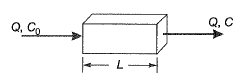Ans: 1.12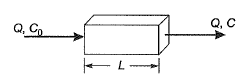For (CMFR) completely mixed flow reactor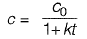For (PFR) plug flow reactor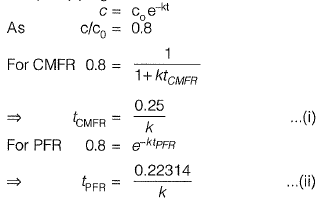v = constant and
L= vt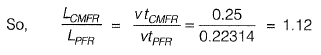Q. 2: A schematic flow diagram of a completely mixed biological reactor with provision for recycling of solids is shown in the figure.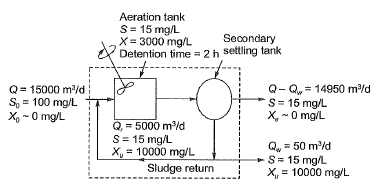Q, Qr Qw = flow rates, m3/d
Xq, X, Xe, Xu = microorganism concentrations (mixed-liquor volatile suspended solids or MLVSS), mg/L
The mean cell residence time (in days, up to one decimal place) is _______ .    [2018 : 2 Marks, Set-II]

Ans: 7.5 days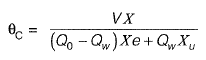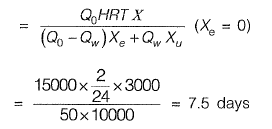Q. 3: The most important type of species involved in the degradation of organic matter in the case of activated sludge process is  [2017 : 1 Mark, Set-II]
(a) autotrophs
(b) heterotrophs
(c) prototrophs
(d) photo-autotrophs

Ans: (b)
Activated sludge process is designed primarily for satisfaction of carbonaceous BOD which is done by heterotrophs.

Q. 4: The wastewater having an organic concentration of 54 mg/l is flowing at a steady rate of 0.8 m3/day through a detention tank of dimensions 2m x 4 m x 2 m. If the contents of the tank are well mixed and the decay contant is 0.1 per day, the outlet concentration (in mg/l, up to one decimal place) is _______ . [2017 : 2 Marks, Set-I]

Ans: 18 mg/l

Q= 0.8 m3/d

Detention time,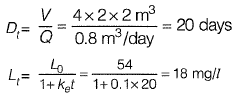Q. 5: Match the items in Group-I with those in Group-ll and choose the right combination    [2016 : 1 Mark, Set-I]

Group-I
P. Activated sludge process
Q. Rising of sludge
R. Conventional nitrification
S. Biological nitrogen removal

Group-ll
1. Nitrifiers and denitrifiers
2. Autotrophic bacteria
3. Heterotrophic bacteria
4. Denitrifiers

(a) P-3, Q-4, R-2, S-1
(b) P-2, Q-3, R-4, S-1
(c) P-2, Q-2, R-4, S-1
(d) P-1, Q-4, R-2, S-3

Ans: (a)

Q. 6: In a wastewater treatment plant, Primary Sedimentation Tank (PST) designed at an overflow rate of 32.5 m3/day/m2 is 32.5 m long, 8.0 m wide and liquid depth of 2.25 m. If the length of the weir is 75 m, the weir loading rate (in m3/day/m) is __________ .   [2015 : 2 Marks, Set-II]
Ans: 112.67m3/d/m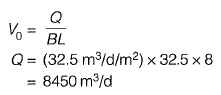Weir length = 75 m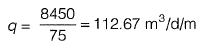Q. 7: Total Kjcldahl Nitrogen ( IKN) concentration (mg/L as N) in domestic sewage is the sum of the concentration of
(a) organic and inorganic nitrogen in sewage
(b) organic nitrogen and nitrate in sewage
(c) organic nitrogen and ammonia in sewage
(d) ammonia and nitrate in sewage [2015 : 1 Mark, Set-I]
Ans: (c)

Kjehldahl Nitrogen = Organic nitrogen + free ammonia.

Q. 8: The amount of C02 generated (in kg ) while completely oxidizing one kg of CH4 to the end products is ___________ . [2014 : 1 Mark, Set-I]
Ans: 2.75kqCO2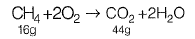⇒ 16 g of CH4 when completely oxidized leads to 44 g of C02

⇒ 1 kg of CH4 when completely oxidized leads to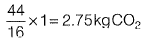Q.9: The dominating microorganisms in an activated sludge process reactor are

[2014 : 1 Mark, Set-II]
(a) aerobic heterotrophs
(b) anaerobic heterotrophs
(d) autotrophs
(d) phototrophs

Ans: (a)

Q. 10: Direction: An activated sludge system (sketched below) is operating at equilibrium with the following information. Waste water related data : flow rate = 500 m3/hour, Influent BOD = 150 mg/L, effluent BOD = 10 mg/L. Aeration tank related data : hydraulic retention time = 8 hours, mean-cell-residence time = 240 hours, volume = 4000 m3, mixed liquor suspended solids = 2000 mg/L.

The mass (in kg/day) of solids wasted from the system is    [2012 : 2 Marks]
(a) 24000
(b) 1000
(c) 800
(d) 33

Ans: (c)

Mean cell recidence time,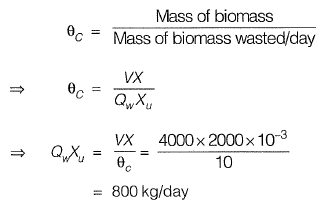Q. 11: Direction: An activated sludge system (sketched below) is operating at equilibrium with the following information. Waste water related data : flow rate = 500 m3/hour, Influent BOD = 150 mg/L, effluent BOD = 10 mg/L. Aeration tank related data : hydraulic retention time = 8 hours, mean-cell-residence time = 240 hours, volume = 4000 m3, mixed liquor suspended solids = 2000 mg/L.

The food-to-biomass (F/M) ratio (in kg BOD per kg biomass per day) for the aeration tank is     [2012 : 2 Marks]
(a) 0.015
(b) 0.210
(c) 0.225
(d) 0.240
Ans: (c)

Given,
Flow rate Q0 = 500 m3/hour
Influent BOD S0 = 150 mg/lit
effluent BOD, = 10 mg/lit.
Hydraulic retention time = 8 hour = 1/3 days
Mean - cell resistance time,
C) = 240 hour = 10 day
M . L . S . S, (X) = 2000 mg/lit.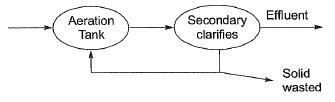Q. 12: Direction: The sludge from the aeration tank of the activated sludge process (ASP) has solids content (by weight) of 2%. This sludge is put in a sludge thickener, where sludge volume is reduced to half. Assume that the amount of solids in the supernatant from the thickener is negligible, the specific gravity of sludge solids is 2.2 and the density of water is 1000 kg/m3

What is the solids content (by weight) of the thickened sludge?  [2011 : 2 Marks]
(a) 3.96%
(b) 4.00%
(c) 4.04%
(d) 4.10%

Ans: (a)

Now,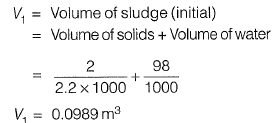Volume of sludge after thickness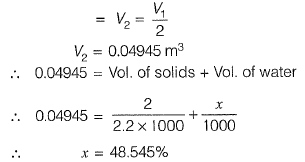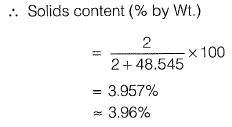Q. 13: Direction: The sludge from the aeration tank of the activated sludge process (ASP) has solids content (by weight) of 2%. This sludge is put in a sludge thickener, where sludge volume is reduced to half. Assume that the amount of solids in the supernatant from the thickener is negligible, the specific gravity of sludge solids is 2.2 and the density of water is 1000 kg/m3.

What is the density of the sludge removed from the aeration tank?   [2011 : 2 Marks]
(a) 990 kg/m3
(b) 1000 kg/m3
(c) 1011 kg/m3
(d) 1022 kg/m3

Ans: (c)
2% of solids + 98% water→ 100% of sludge letx be the density of sludge.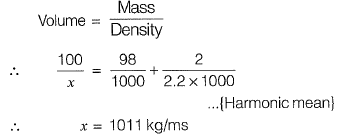Offer running on EduRev: Apply code STAYHOME200 to get INR 200 off on our premium plan EduRev Infinity!

67 docs

,

,

,

,

,

,

,

,

,

,

,

,

,

,

,

,

,

,

,

,

,

;